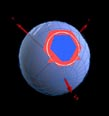HomeSDSS MirrorsDocumentationHTMCfunBASESkyServerCasJobsHelpTeamSkyServer.org - HTM

#The Hierarchical Triangular Mesh

## Programmers Quick Guide

This is not an exhaustive guide but wishes to give quick access to the most salient features of the HTM libraries in the minimum time. Further detailed information such as Javadocs and docs++ are available for download or online from the the implementaion page.

Below two complete programms are listed in Java and in C++ which utilise the different HTM classes to perform a lookup and an intersection.

### Lookup an ID

This is the most basic operation in HTM. The lookup applications both give access to this funtionality as described in the user guide . This code is in the download - in java it is in the src/edu/jhu/htmExamples directory, in the c++ it is in the app directory.

The inputs to lookup are a level number and either an RA,Dec or x,y,z coordinate. Assuming you have the code and already got it to compile then if you wanted to use this function in your code you would simply have something like :

#### in java :

```
import edu.jhu.htm.core.*;

public class Look {

public static void main(String[] args) {

int arg=0;

int level       = Integer.parseInt(args[arg++]);

double RA       = Double.parseDouble(args[arg++]);

double Dec      = Double.parseDouble(args[arg++]);

// create the level index required

HTMindex si = new HTMindexImp(level);

// lookup hte name by RA dec.

try {

String htmName    = si.lookup(RA,Dec);

// actaully lookupid is more efficient

//if you dont want the name

long htmIdNumber= si.nameToId(htmName);

System.out.println(htmIdNumber+" "+htmName);

} catch (HTMException e) {

System.err.println("could not do look "+e);

}

}

}

```

#### in c++ :

```
#include

#include "SpatialVector.h"

#include "SpatialIndex.h"

int

main(int argc, char *argv[]) {

int args=1;

size_t level = atoi(argv[args++]);

float64 ra = atof(argv[args++]);

float64 dec = atof(argv[args++]);

// create level index required

SpatialIndex* htm = new SpatialIndex(level);

// lookup id by ra dec

uint64 id = htm->idByPoint(ra,dec);

PRINTID(id); // problems with format for uint64 on different platforms

// so we use this macro to print them

printf(" %s\n",htm->nameById(id));

}

```

### Perform a complex intersection

Domain files allow arbirarily complex intersections to be performed using HTM. The User Guide describes the intersect application. Here however I would like to look at a simpler intersection with a rectangular field such as provided by HtmTools.jsp .

More complex operations on HTM must be done using vectors rather than RA and Dec, however the handy Vevtor3d(SpatialVector in version2.1) class lets you convert easily between they two. Also in Version 3.0 we have new geometry classes which can make shapes using RAs and Decs and return domains or convexes.

When we do an intersection with the HTM the result is a HTMRange - so we do not get back a list of HTMs directly rather a set of ranges of HTMs which make up the full set. Hence rather if your intersect was going to return N121 N122 N123 N124 instead you would get a range N121,N124. The HtmRange class allows one to iterate ove ther ranges geting back each low,high pair. The HtmRangeIterator expands the the set to all the Ids.

This is all demonstrated in the following short program to intersect with a rectangle.

#### in Java

```

import edu.jhu.htm.core.*;

import edu.jhu.htm.geometry.*;

import java.util.*;

public class RecIntersect {

/** input ra,dec for top corner and ra,dec for bottom corner */

public static void main(String[] args) throws HTMException {

int arg                 = 0;

int level               = Integer.parseInt(args[arg++]);

double[] inp 		= new double;

// asume 2 corners come ra dec ra dec

for (int c = 0 ; c < 4 ; c++ ) {

inp[c]       = Double.parseDouble(args[arg++]);

}

// create the level index required

HTMindex si = new HTMindexImp(level);

// create a Rectangle

Rect rec = new Rect(inp,inp,inp,inp);

// get the domain for intersection

Domain dom = rec.getDomain();

dom.setOlevel(level);// only want triangles at this level

// we need a range to recieve the result in

HTMrange range = new HTMrange();

// do the intersect the boolean is for varlenght ids or not

dom.intersect((HTMindexImp)si,range,false);

System.out.println(range);

// but I might want to see all the htm numbers

// I can iterate through them and have them

// The boolean gives them as names rather than ids

Iterator iter = new HTMrangeIterator(range,true);

while (iter.hasNext()) {

System.out.println(iter.next());

}

}

}

```

#### in c++ :

```
#include

#include "SpatialVector.h"

#include "SpatialIndex.h"

#include "RangeConvex.h"

#include "HtmRange.h"

#include "HtmRangeIterator.h"

int

main(int argc, char *argv[]) {

int args              =1;

size_t level          = atoi(argv[args++]);

float64 ra            = 0;

float64 dec           = 0;

SpatialVector* cnrs;

// assume 4 points and read them in

for (int c = 0 ; c < 4 ; c++ ) {

ra      = atof(argv[args++]);

dec     = atof(argv[args++]);

cnrs[c] = new SpatialVector(ra,dec);

}

// create level index required

SpatialIndex* si = new SpatialIndex(level);

// lookup id by ra dec

// Create convex to represent the rectangle

RangeConvex* conv = new  RangeConvex(cnrs,cnrs,cnrs,cnrs);

conv->setOlevel(level);// only want triangles at this level

// we need a range to recieve the result in

HtmRange* range = new HtmRange();

// do the intersect the boolean is for varlenght ids or not

conv->intersect(si,range,false);

cout << *range<< endl;

// but I might want to see all the htm numbers

// I can iterate through them and have them

HtmRangeIterator* iter = new HtmRangeIterator(range);

char* buf = (char*)malloc(128);

while (iter->hasNext()) {

// next gives the numeric ID nextSymbolic converts it to a name

printf(" %s\n",iter->nextSymbolic(buf));

}

}

```

William O'Mullane
Last Modified :Tuesday, December 26, 2006 at 3:52:09 PM , \$Revision 1.4 \$